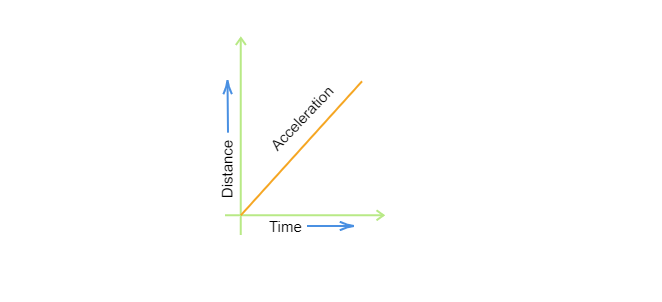# Slope of a velocity-time graph giveshe following cases of motions, the distance moved and the magnitude of displacement are equal?$(a)$. If the car is moving on straight road$(b)$. If the car is moving in circular path$(c)$. The pendulum is moving to and fro$(d)$. The earth is revolving around the sun

The slope of the velocity-time graph shows acceleration.So, option $(c)$ is correct.

Updated on: 10-Oct-2022

19 Views